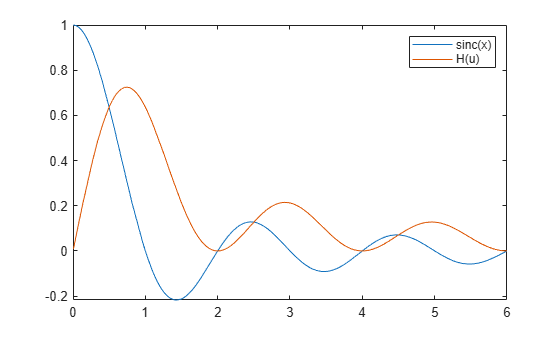htrans

Hilbert transform

Description

example

H = htrans(f) returns the Hilbert transform of symbolic function f. By default, the independent variable is t and the transformation variable is x.

example

H = htrans(f,transVar) uses the transformation variable transVar instead of x.

example

H = htrans(f,var,transVar) uses the independent variable var and the transformation variable transVar instead of t and x, respectively.

• If all input arguments are arrays of the same size, then htrans acts element-wise.

• If one input is a scalar and the others are arrays of the same size, then htrans expands the scalar into an array of the same size.

• If f is an array of symbolic expressions with different independent variables, then var must be a symbolic array with elements corresponding to the independent variables.

Examples

collapse all

Compute the Hilbert transform of sin(t). By default, the transform returns a function of x.

syms t;
f = sin(t);
H = htrans(f)
H = $-\mathrm{cos}\left(x\right)$

Compute the Hilbert transform of the sinc(x) function, which is equal to sin(pi*x)/(pi*x). Express the result as a function of u.

syms f(x) H(u);
f(x) = sinc(x);
H(u) = htrans(f,u)
H(u) =

$-\frac{\frac{\mathrm{cos}\left(\pi u\right)}{u}-\frac{1}{u}}{\pi }$

Plot the sinc function and its Hilbert transform.

fplot(f(x),[0 6])
hold on
fplot(H(u),[0 6])
legend('sinc(x)','H(u)')Create a sine wave with a positive frequency in real space.

syms A x t u;
assume([x t],'real')
y = A*sin(2*pi*10*t + 5*x)
y = $A \mathrm{sin}\left(5 x+20 \pi t\right)$

Apply a –90-degree phase shift to the positive frequency component using the Hilbert transform. Specify the independent variable as t and the transformation variable as u.

H = htrans(y,t,u)
H = $-A \mathrm{cos}\left(5 x+20 \pi u\right)$

Now create a complex signal with negative frequency. Apply a 90-degree phase shift to the negative frequency component using the Hilbert transform.

z = A*exp(-1i*10*t)
z = $A {\mathrm{e}}^{-10 t \mathrm{i}}$
H = htrans(z)
H = $A {\mathrm{e}}^{-10 x \mathrm{i}} \mathrm{i}$

Create a real-valued signal $\mathit{f}\left(\mathit{t}\right)$ with two frequency components, 60 Hz and 90 Hz.

syms t f(t) F(s)
f(t) = sin(2*pi*60*t) + sin(2*pi*90*t)
f(t) = $\mathrm{sin}\left(120 \pi t\right)+\mathrm{sin}\left(180 \pi t\right)$

Calculate the corresponding analytic signal $\mathit{F}\left(\mathit{s}\right)$ using the Hilbert transform.

F(s) = f(s) + 1i*htrans(f(t),s)
F(s) = $\mathrm{sin}\left(120 \pi s\right)+\mathrm{sin}\left(180 \pi s\right)-\mathrm{cos}\left(120 \pi s\right) \mathrm{i}-\mathrm{cos}\left(180 \pi s\right) \mathrm{i}$

Calculate the instantaneous frequency of $\mathit{F}\left(\mathit{s}\right)$ using

${f}_{instant}\left(s\right)=\frac{1}{2\pi }\frac{d\varphi \left(s\right)}{ds},$

where $\varphi \left(s\right)=\mathrm{arg}\left[F\left(s\right)\right]$ is the instantaneous phase of the analytic signal.

InstantFreq(s) = diff(angle(F(s)),s)/(2*pi);
assume(s,'real')
simplify(InstantFreq(s))
ans = $75$

Input Arguments

collapse all

Input, specified as a symbolic expression, symbolic function, symbolic vector, or symbolic matrix.

Independent variable, specified as a symbolic variable, symbolic vector, or symbolic matrix. This variable is usually in the time domain. If you do not specify the variable, then htrans uses t by default. If f does not contain t, then htrans uses the function symvar to determine the independent variable.

Transformation variable, specified as a symbolic variable, symbolic vector, or symbolic matrix. This variable is in the same domain as var. If you do not specify the variable, then htrans uses x by default. If x is the independent variable of f, then htrans uses the transformation variable v.

Output Arguments

collapse all

Hilbert transform or harmonic conjugate of the input function f. The output H is a function of the variable specified by transVar.

When htrans cannot transform the input function, it returns an unevaluated call. To return the original expression, apply the inverse Hilbert transform to the output by using ihtrans.

collapse all

Hilbert Transform

The Hilbert transform H = H(x) of the expression f = f(t) with respect to the variable t at point x is

$H\left(x\right)=\frac{1}{\pi }\text{p}\text{.v}\text{.}\underset{-\infty }{\overset{\infty }{\int }}\frac{f\left(t\right)}{x-t}dt.$

Here, p.v. represents the Cauchy principal value of the integral. The function f(t) can be complex, but t and x must be real.

Tips

• To compute the inverse Hilbert transform, use ihtrans. The Hilbert transform of a function is equal to the negative of its inverse Hilbert transform.

• For a signal in the time domain, the Hilbert transform applies a –90-degree phase shift to positive frequencies of the corresponding Fourier components. It also applies a 90-degree phase shift to negative frequencies.

• For a real-valued signal a, the Hilbert transform b = htrans(a) returns its harmonic conjugate b. The real signal a = real(z) and its Hilbert transform b = imag(z) form the analytic signal z = a + 1i*b.